# Insertion Sort Algorithm | Example | Time Complexity

## Insertion Sort-

• Insertion sort is an in-place sorting algorithm.
• It uses no auxiliary data structures while sorting.
• It is inspired from the way in which we sort playing cards.

## How Insertion Sort Works?

Consider the following elements are to be sorted in ascending order-

6, 2, 11, 7, 5

Insertion sort works as-

Firstly,

• It selects the second element (2).
• It checks whether it is smaller than any of the elements before it.
• Since 2 < 6, so it shifts 6 towards right and places 2 before it.
• The resulting list is 2, 6, 11, 7, 5.

Secondly,

• It selects the third element (11).
• It checks whether it is smaller than any of the elements before it.
• Since 11 > (2, 6), so no shifting takes place.
• The resulting list remains the same.

Thirdly,

• It selects the fourth element (7).
• It checks whether it is smaller than any of the elements before it.
• Since 7 < 11, so it shifts 11 towards right and places 7 before it.
• The resulting list is 2, 6, 7, 11, 5.

Fourthly,

• It selects the fifth element (5).
• It checks whether it is smaller than any of the elements before it.
• Since 5 < (6, 7, 11), so it shifts (6, 7, 11) towards right and places 5 before them.
• The resulting list is 2, 5, 6, 7, 11.

As a result, sorted elements in ascending order are-

2, 5, 6, 7, 11

## Insertion Sort Algorithm-

Let A be an array with n elements. The insertion sort algorithm used for sorting is as follows-

```for (i = 1 ; i < n ; i++)
{
key = A [ i ];
j = i - 1;
while(j > 0 && A [ j ] > key)
{
A [ j+1 ] = A [ j ];
j--;
}
A [ j+1 ] = key;
}```

Here,

• i = variable to traverse the array A
• key = variable to store the new number to be inserted into the sorted sub-array
• j = variable to traverse the sorted sub-array

## Insertion Sort Example-

Consider the following elements are to be sorted in ascending order-

6, 2, 11, 7, 5

The above insertion sort algorithm works as illustrated below-

### Step-01: For i = 1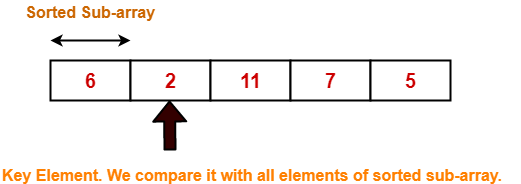### Step-02: For i = 2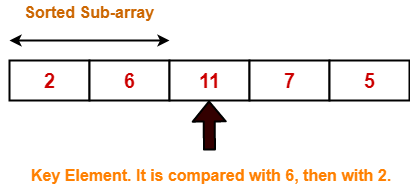### Step-03: For i = 3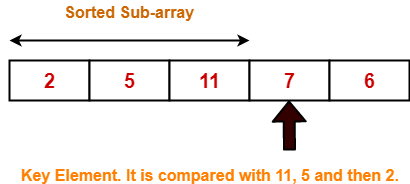2 5 11 7 6 For j = 2; 11 > 7 so A = 11 2 5 11 11 6 For j = 1; 5 < 7 so loop stops and A = 7 2 5 7 11 6 After inner loop ends

Working of inner loop when i = 3

### Step-04: For i = 4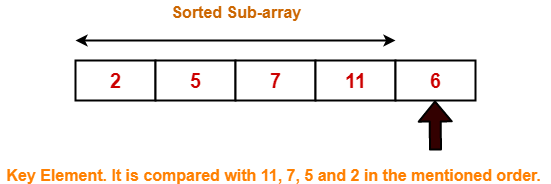Loop gets terminated as ‘i’ becomes 5. The state of array after the loops are finished-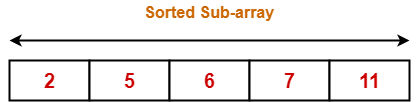With each loop cycle,

• One element is placed at the correct location in the sorted sub-array until array A is completely sorted.

## Time Complexity Analysis-

• Selection sort algorithm consists of two nested loops.
• Owing to the two nested loops, it has O(n2) time complexity.

 Time Complexity Best Case n Average Case n2 Worst Case n2

## Space Complexity Analysis-

• Selection sort is an in-place algorithm.
• It performs all computation in the original array and no other array is used.
• Hence, the space complexity works out to be O(1).

## Important Notes-

• Insertion sort is not a very efficient algorithm when data sets are large.
• This is indicated by the average and worst case complexities.
• Insertion sort is adaptive and number of comparisons are less if array is partially sorted.

To gain better understanding about Insertion Sort Algorithm,

Watch this Video Lecture

Next Article- Merge Sort

### Other Popular Sorting Algorithms-

Get more notes and other study material of Design and Analysis of Algorithms.

Watch video lectures by visiting our YouTube channel LearnVidFun.

SummaryArticle Name
Insertion Sort Algorithm | Example | Time Complexity
Description
Insertion Sort is a famous approach to sorting. Insertion Sort Algorithm with Example is given. Insertion Sort Algorithm Time Complexity is O(n2). Insertion Sort Algorithm Space Complexity is O(1).
Author
Publisher Name
Gate Vidyalay
Publisher Logo